# CS 61B | Part 8 | Tries (Prefix), Range Searching and Multi-Dimensional Data

## Tries

### Reflection

In terms of the ADTs Set and Map, let’s recall the runtimes for both of these:

• Balanced Search Tree:
• contains(x): $\Theta(\log{N})$
• add(x): $\Theta(\log{N})$
• Resizing Separate Chaining Hash Table:
• contains(x): $\Theta(1)$ (assuming even spread)
• add(x): $\Theta(1)$ (assuming even spread and amortized)

Question: Can we do even better than this? That could depend on the nature of our problem. What if we knew a special characteristic of the keys?

Answer: Yes, for example if we always know our keys are ASCII characters then instead of using a general-purpose HashMap, simply use an array where each character is a different index in our array:

The value R represents the number of possible characters (e.g. 128 for ASCII). So, by knowing the range of our keys as well as what types of values they will be we have a simple and efficient data structure.

A key takeaway is that we can often improve a general-purpose data structure when we add specificity to our problem, often by adding additional constraints. For example, we improved our implementation of HashMap when we restricted the keys to only be ASCII character, creating extremely efficient data structure.

### Definition

The Trie is a specific implementation for Sets and Maps that is specialized for strings (like hash table is specialized for the non-comparable).

It is a short for Retrieval tree, but almost everyone pronounces it as “try”. The inventor Edward Fredkin suggested it be pronounced as “tree”.

Tries are a very useful data structure used in cases where keys can be broken into “characters” and share prefixes with other keys (e.g. strings).

With the previous implementations BST and RST Hash Table, we may have the following structures: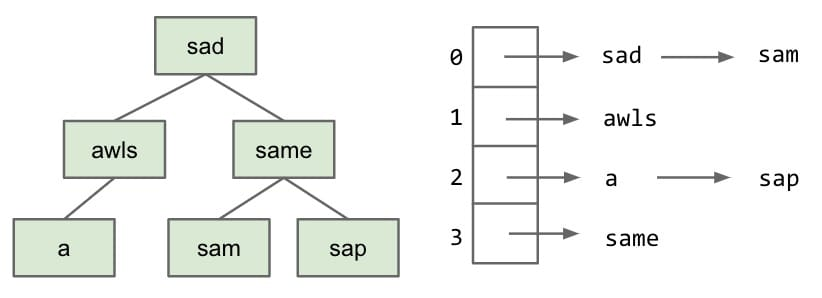Key ideas:

• Every node stores only one letter.
• Nodes can be shared by multiple keys.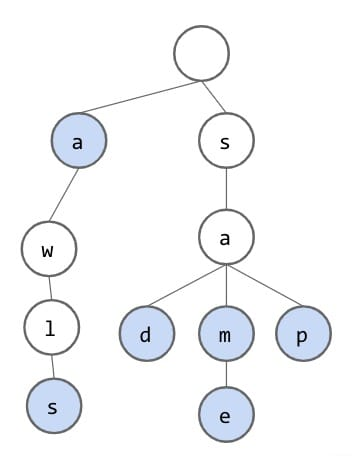An important observation to make is that most of these words share the same prefixes.

To check if the trie contains a key, walk down the tree from the root along the correct nodes.

Since we are going to share nodes, we must figure out some way to represent which strings belong in our set and which don’t. To search, we will traverse our trie and compare to each character. There are only two cases when we would not be able to find a string:

• Hit an unmarked node or the final node is not marked (not the ending node).
• Fall off the tree (no node matching the current character).

A trie can be used as a map too.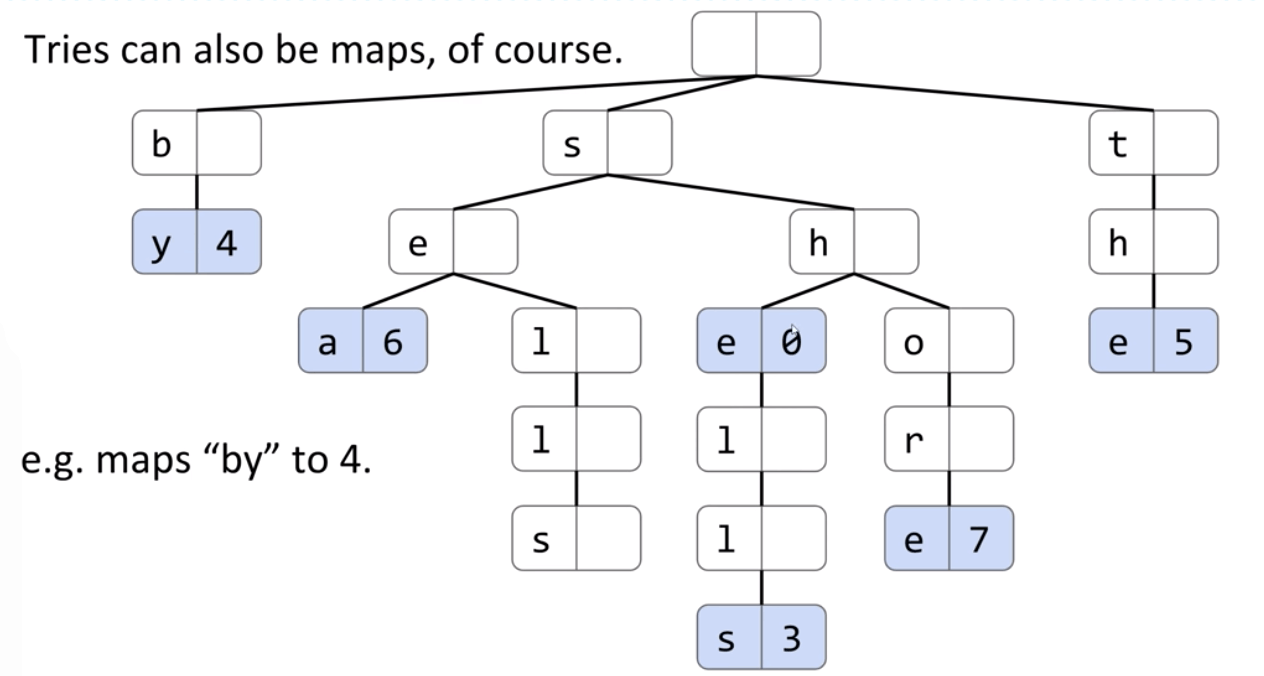### Implementation

#### Approach 1

DataIndexedCharMap is for a node accessing its children. For example, node a can access node d by “d”, node m by “m”, and node p by “p”.We can make an important observation: Each link corresponds to a character if and only if that character exists. Therefore, we can remove the Node’s character variable and instead base the value of the character from its position in the parent DataIndexedCharMap.

Note: In real implementation, contains(char) and contains(String) are different. In this case, we’d better use String.

Problem: If we use a DataIndexedCharMap to track children, every node has $R$ links.

Observation: The letter stored inside each node is actually redundant. So we can remove from the representation.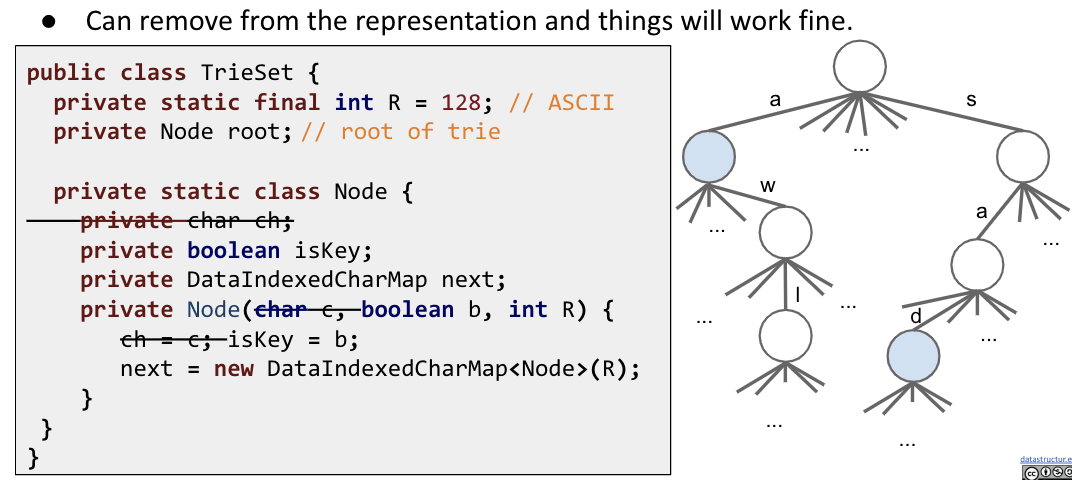Performance in Terms of N

Given a Trie with $N$ keys. What are the runtimes of add and contains? $\Theta(1)$.

Runtimes independent of number of keys. It doesn’t matter how many items we have in our trie.

Or in terms of $L$, the length of the key: $\Theta(L)$.

#### Approach 2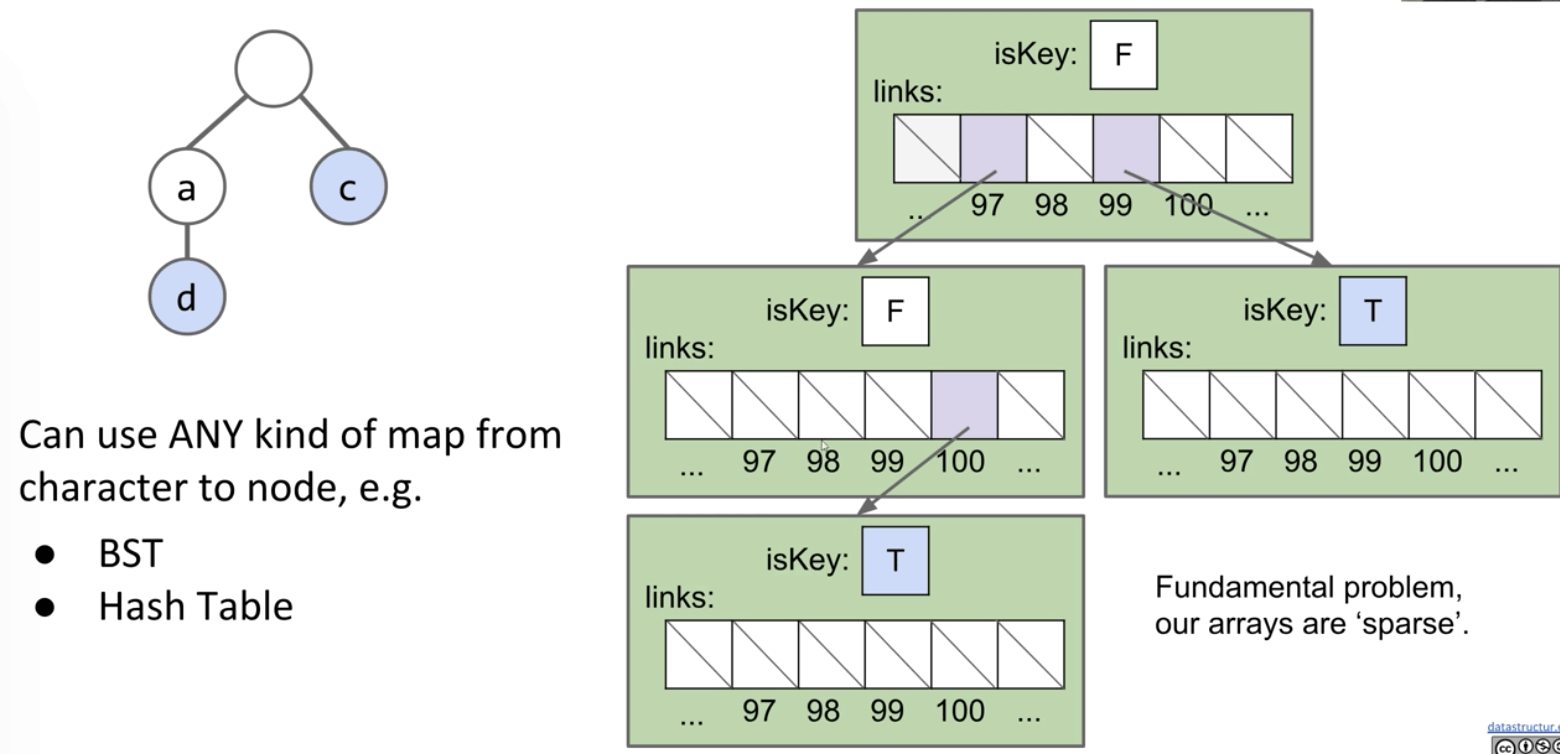Alternate Idea #1: Hash Table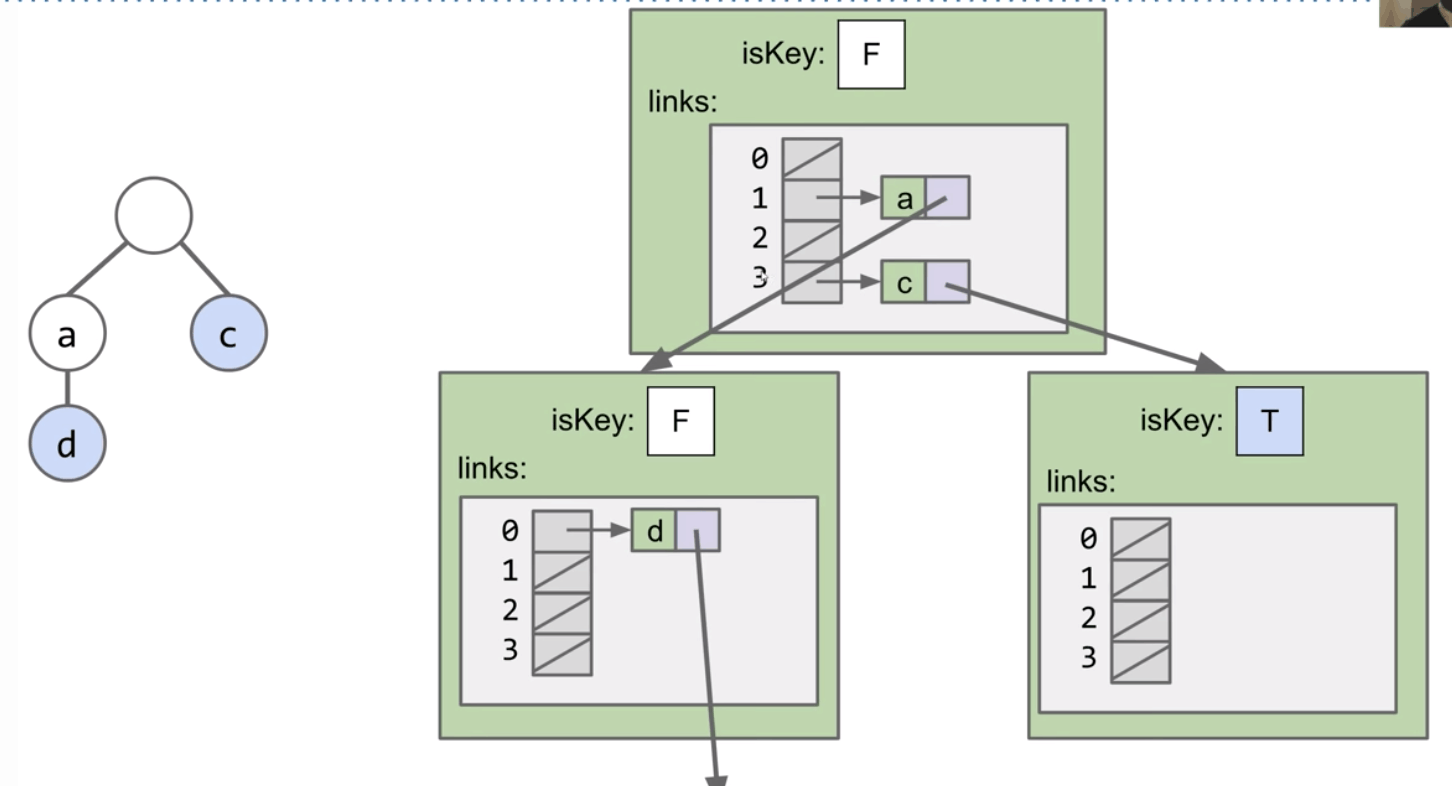Alternate Idea #2: BST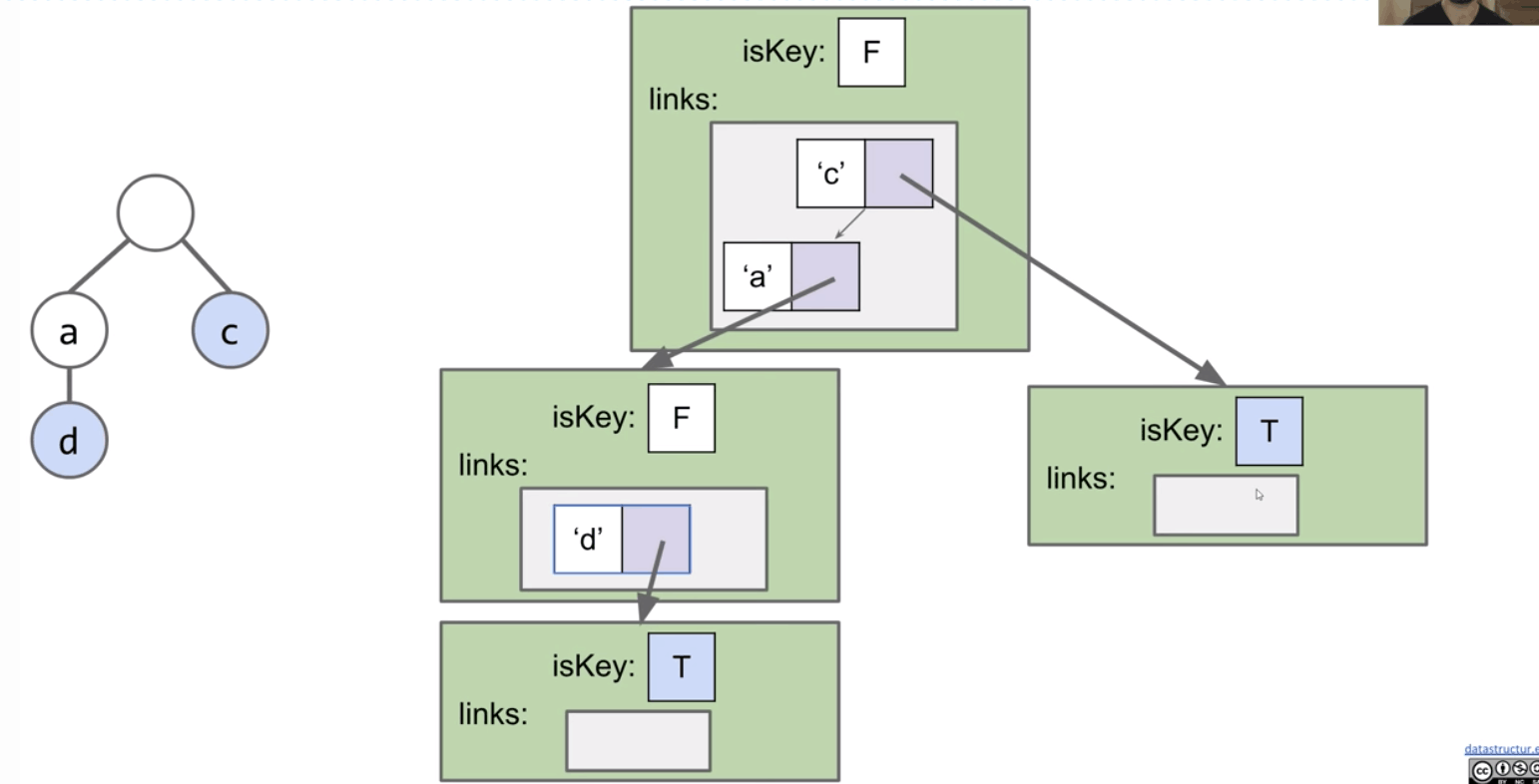So, when we implement a Trie, we have to pick a map to our children.

Now we have:

• DataIndexedCharMap: Very fast, but memory hungry.
• Hash Table: Almost as fast, uses less memory.
• Balanced BST: A little slower than Hash Table, uses similar amount of memory.

Let’s look into the details:

• DataIndexedCharMap
• Space: 128 links per node.
• Runtime: $\Theta(1)$
• BST
• Space: $C$ links per node, where $C$ is the number of children.
• Runtime: $O(\log{R})$, where $R$ is the size of alphabet.
• Hash Table
• Space: $C$ links per node.
• Runtime: $O(R)$. (if all keys all mapping to a bucket)

Note: Cost per link is higher in BST and Hash Table.

However, since $R$ is fixed (e.g. 128), we can think of all three as $\Theta(1)$.

You would have to do computational experiments to see which is best for your application.

### String Operations

Theoretical asymptotic speed improvement is nice, but the main appeal of tries is their ability to efficiently support string specific operations like prefix matching.

• Finding all keys that match a given prefix: keysWithPrefix("sa")
• Finding longest prefix of: longestPrefixOf("sample")

#### collect()

Given an algorithm for collecting all the keys in a Trie.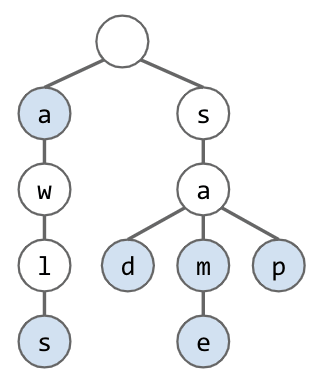collect() returns [“a”, “awls”, “sad”, “sam”, “same”, “sap”]

collect():

• Create an empty list of returns x
• For character c in root.next.keys():
• Call colHelp(c, root.next.get(c))
• Return x

colHelp(String s, List x, Node n):

• If n.isKey == true, then x.add(s).
• For character c in n.next.keys(): Call colHelp again.

#### keysWithPrefix()

Example: keysWithPrefix(“sa”) is [“sad”, “sam”, “same”, “sap”].

• Find the node a corresponding to the prefix string pf.
• Create an empty list x.
• For character c in a.next.keys():
• Call colHelp("sa" + c, x, a.next.get(c)).

#### Autocomplete

Example: When we type “how are” into Google, we get 10 results.

One way to do this is to create a Trie-Based Map from strings to values.

• Value: Represents how important Google thinks that string is.
• Can store billions of strings efficiently since they share nodes.
• When a user types in a string “hello”, we:
• Call keysWithPrefix("hello").
• Return the 10 strings with the highest value.

More Efficient:

If we enter a short string, the number of keys with the appropriate prefix will be too big.

Solution: Each node stores its own value, as well as the value of its best substring.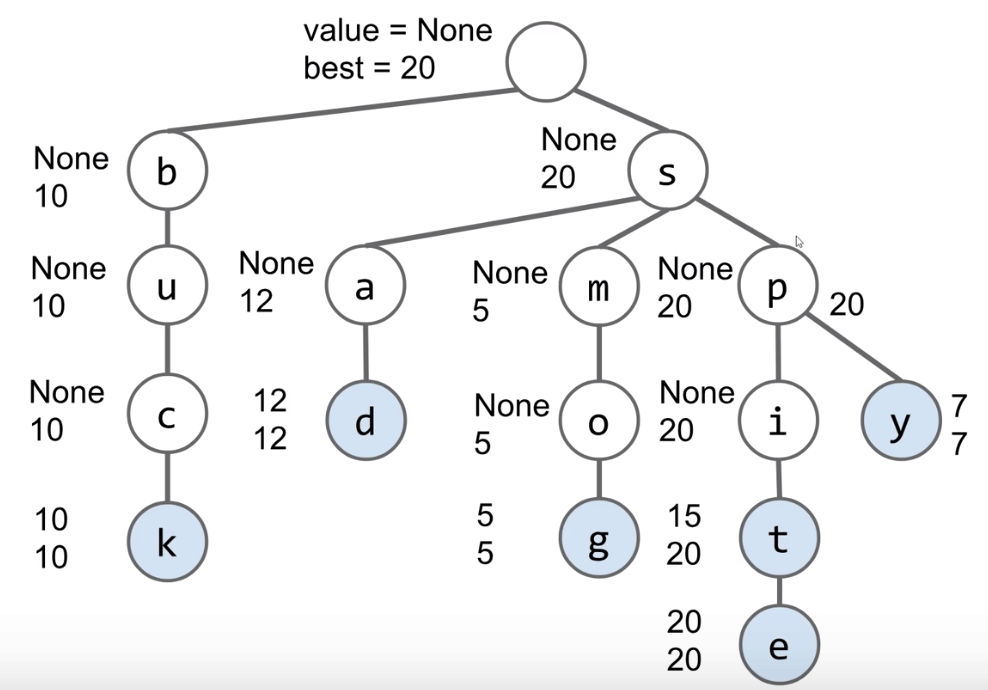Search will consider nodes in order of “best”.

• Consider sp before sm (since the value of node p is 20).
• Can stop when top 3 matches are all better than best remaining.

Hint: Use a PQ.

Even More Efficient:

Can also merge nodes that are redundant!

• This version of trie is known as a radix tree or radix trie.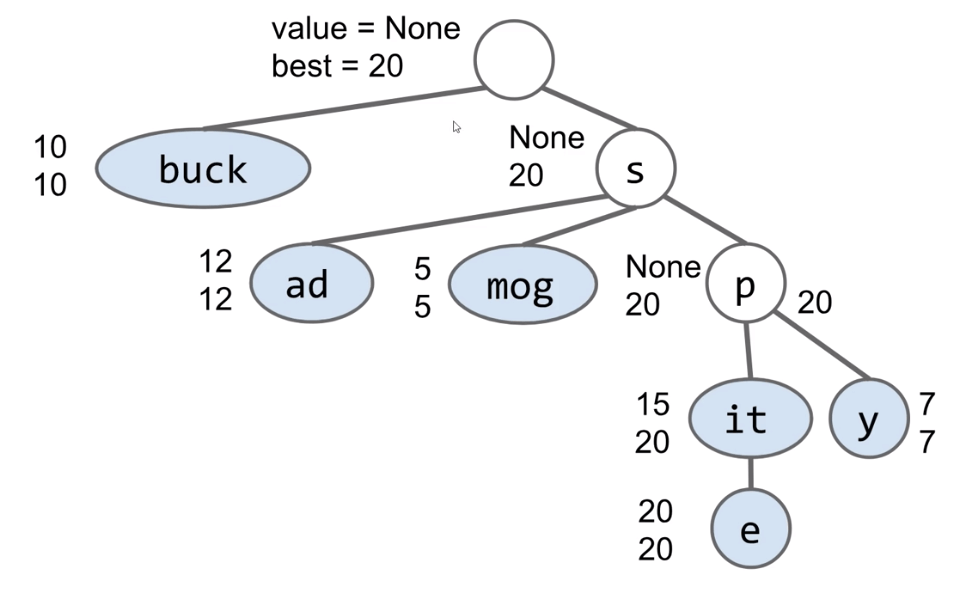Bottom line: Data structures interact in beautiful and important ways!

## Ex (Guide)

### C level

#### Ex 1 (TST)

Problem 5 from Princeton’s Spring 2008 final.

A ternary search tree (also called a prefix tree) is a special trie data structure where the child nodes of a standard trie are ordered as a binary search tree.

Unlike tries where each node contains 26 pointers for its children, each node in a ternary search tree contains only 3 pointers:

• The left pointer must point to a node whose character value is less than the current node.
• The right pointer must point to a node whose character is greater than the current node.
• The equal pointer points to the next character in the word.

Problem: Consider the following TST, where the black nodes correspond to strings in the TST.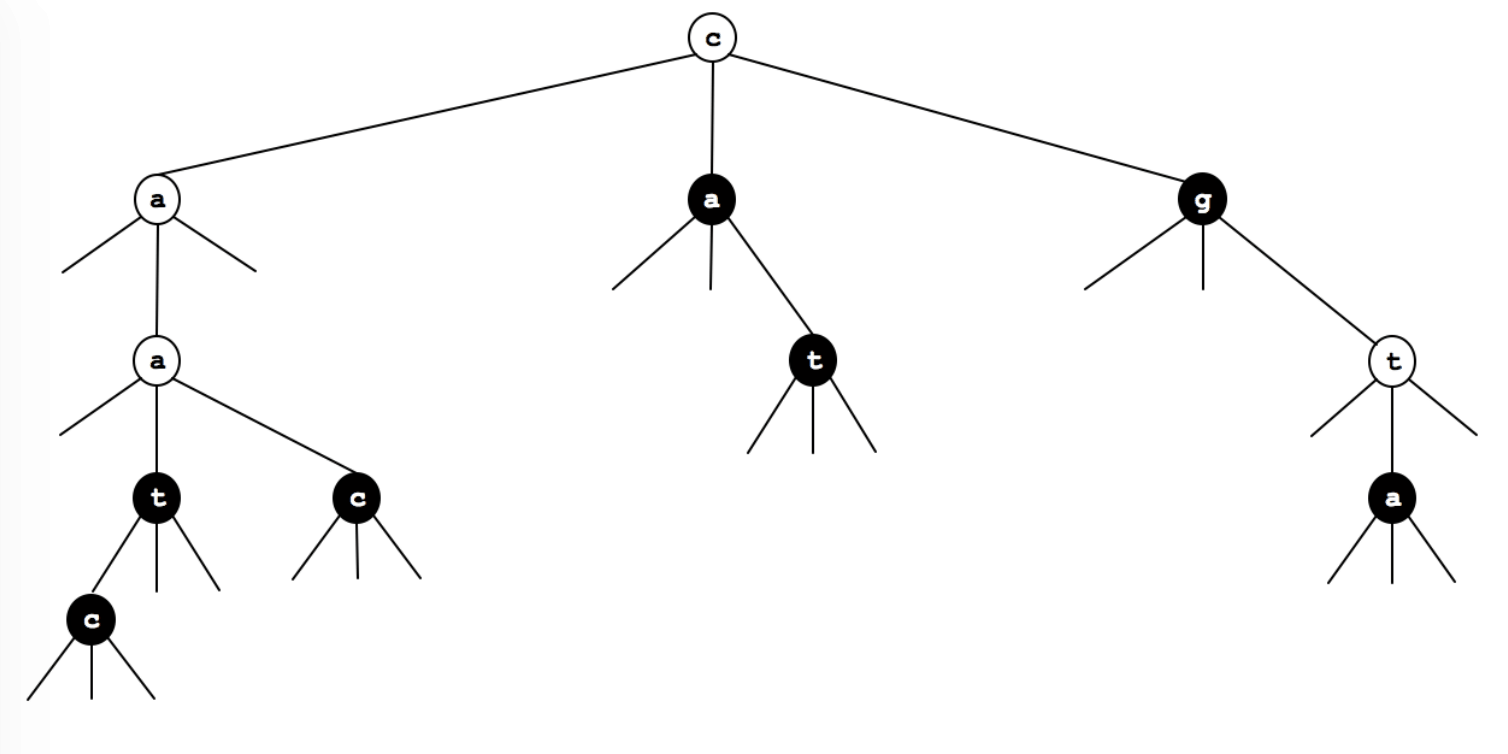(a) Which 7 strings (in alphabetical order) are in the TST?

aac, aat, ac, ca, ct, g, ta

(b) Draw the results of adding the following strings into the TST above: cgt, aaca, tt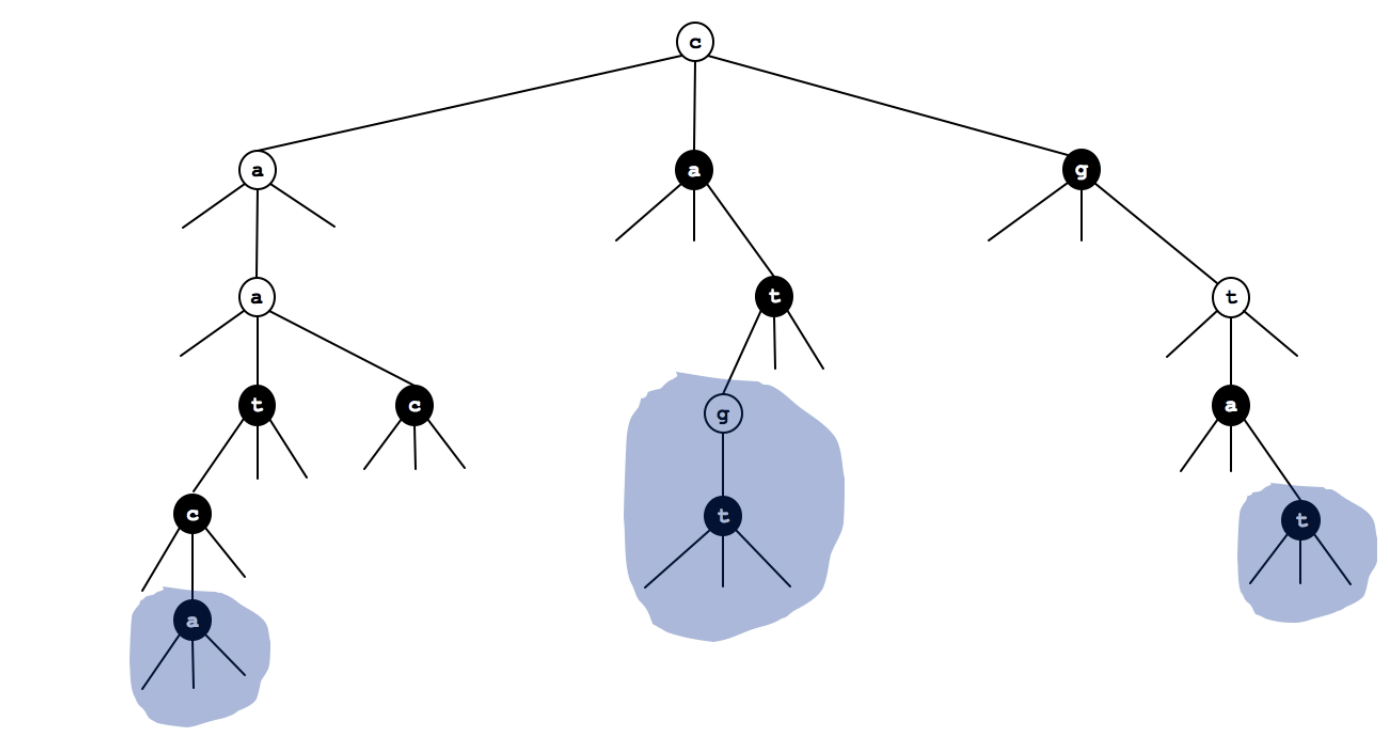#### Ex 2 (TST)

Problem 8 from Princeton’s Spring 2011 final.

Consider the following ternary search trie, with string keys and integer value.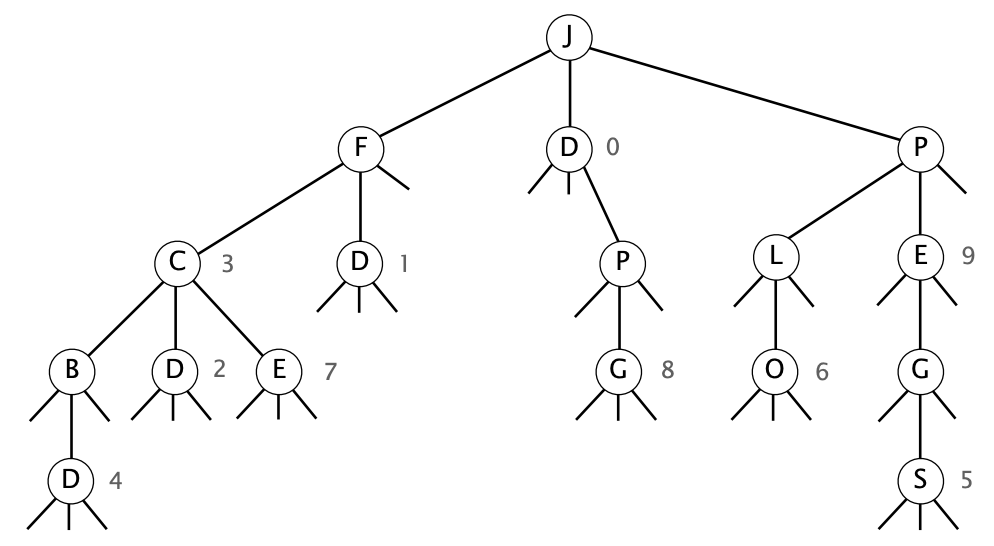Circle which one or more of the following strings are keys in the TST.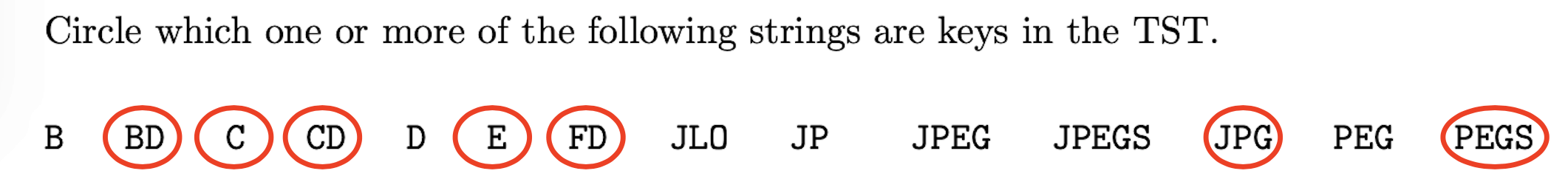### B level

Throughout, we define $R$ as the size of the alphabet, and $N$ as the number of items in a trie.

#### Ex 1 (Single Character String)

When looking for a single character string in a Trie, what is the worst case time to find that string in terms of $R$ and $N$?

$\Theta(1)$

#### Ex 2 (Why TST?)

Problem 5 from Princeton’s Fall 2009 final.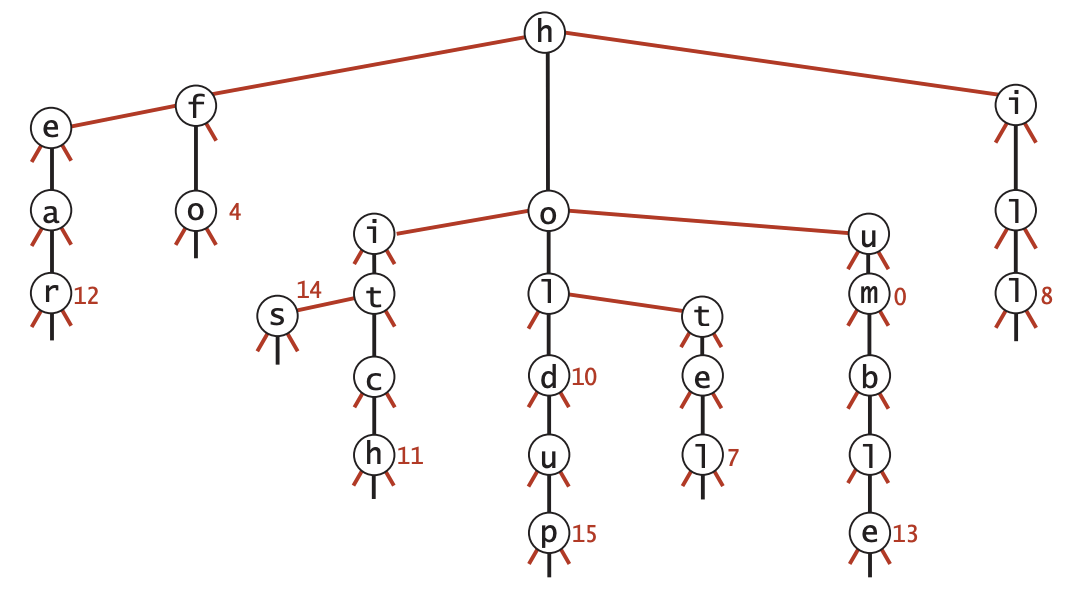(a) List (in alphabetical order) the set of strings that were inserted.

ear, fo, his, hitch, hold, holdup, hotel, hum, humble, ill.

(c) List two compelling reasons why a programmer would use a TST instead of a red-black tree.

• Faster, especially for search miss.
• Support character-based operations such as prefix match (autocomplete), longest prefix, and wildcard match.

#### Ex 3 (Height of TST)

Problem 9 from Princeton’s Fall 2012 final.

Suppose that the following set of 11 strings are inserted into a TST (in some order).

CANNON, CAP, CHARTER, CLOISTER, COLONIAL, COTTAGE,
IVY, QUAD, TERRACE, TIGER, TOWER

(a) Suppose that the strings are inserted into the TST in alphabetical order. What is the height of the resulting TST?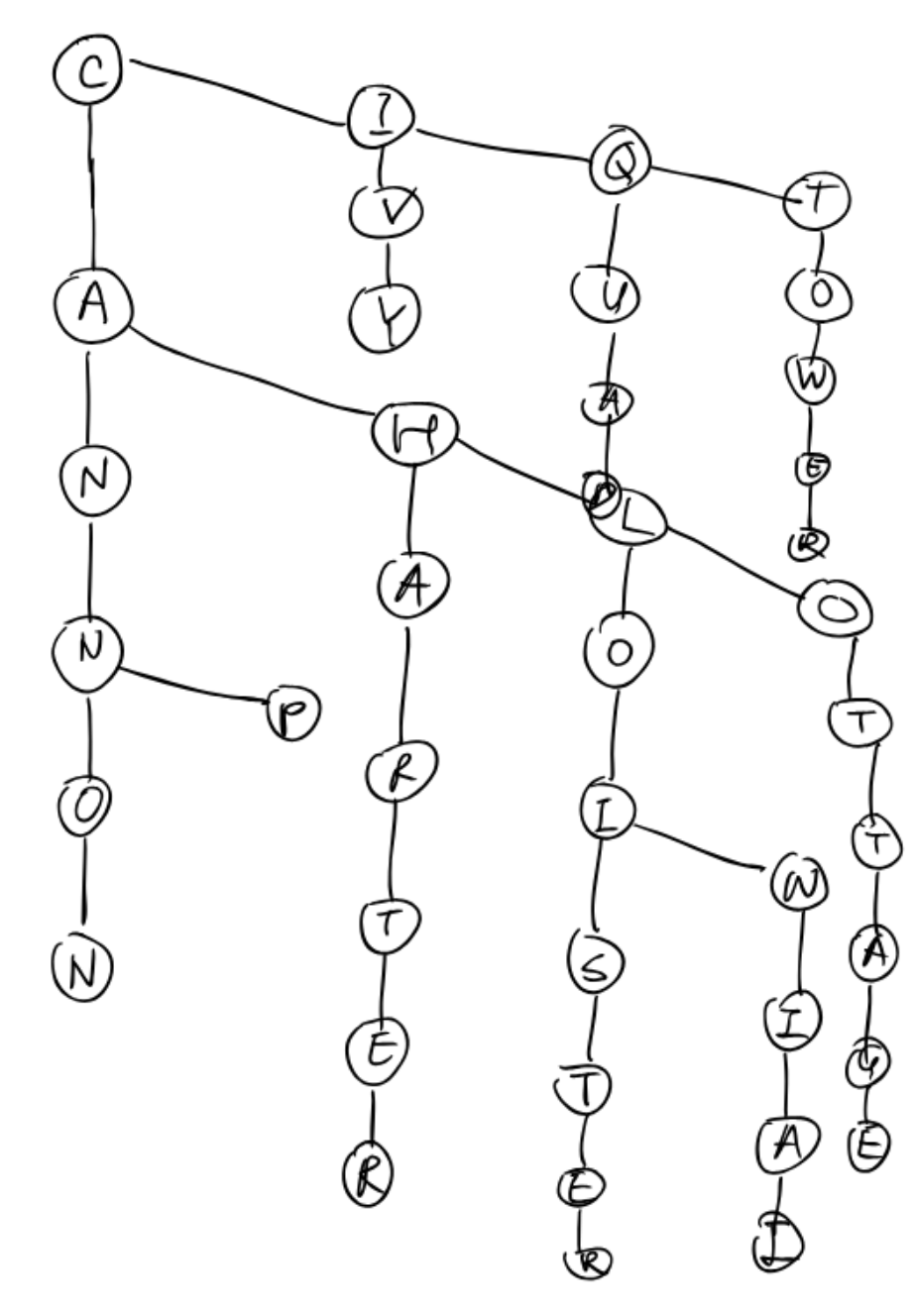The height is 9.

(b) Suppose that the strings are inserted into the TST in an order which minimizes the height. What is the height then?

Since COLONIAL and CLOISTER each contains 8 characters, at least one of them will end up at depth 8 (height = 7) or higher. To control the height, we can add COLONIAL and CLOISTER before other items.

#### Ex 4 (Height of TST)

Problem 1 from Hug’s Fall 2013 final.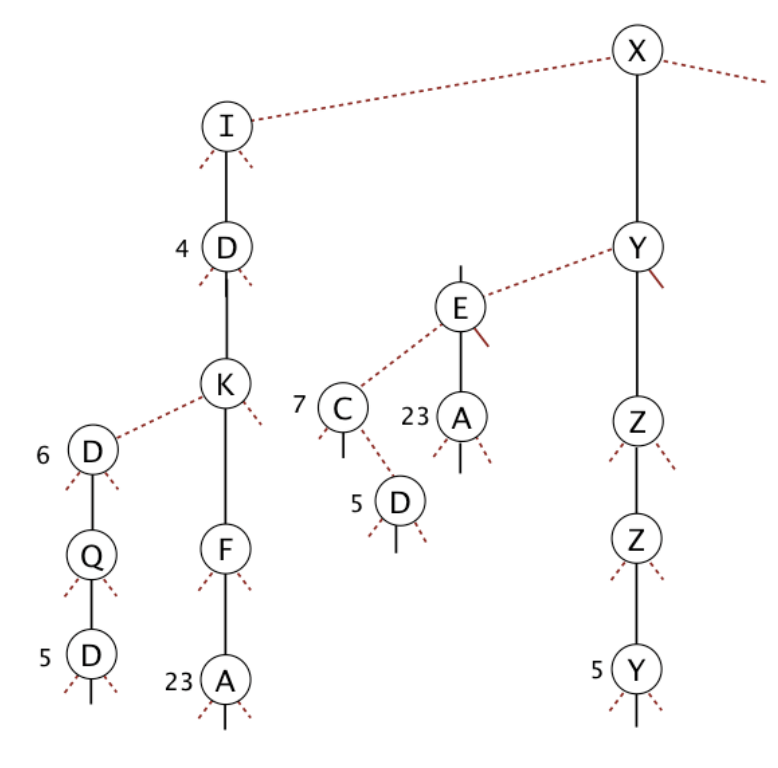(a) List the 8 strings in the TST in alphabetical order.

ID, IDD, IDDQD, IDKFA, XC, XD, XEA, XYZZY.

(b) Give an example of a minimum length string that will increase the height of the TST.

IDAAAA or XDAAAA or XCAAAA.

### A level

#### Ex 1 (Questions)

Problem 9 from Hug’s Fall 2014 midterm 2.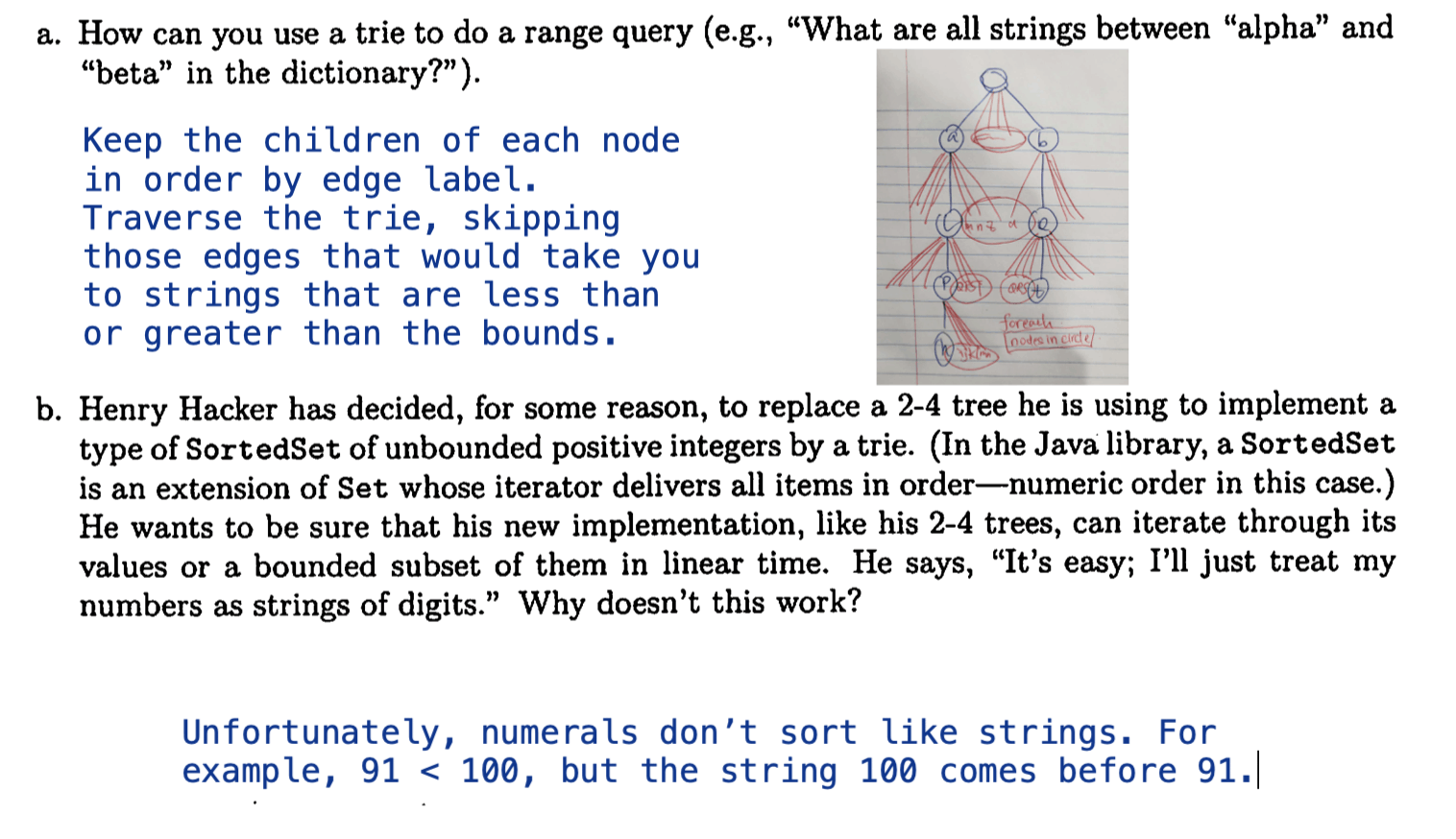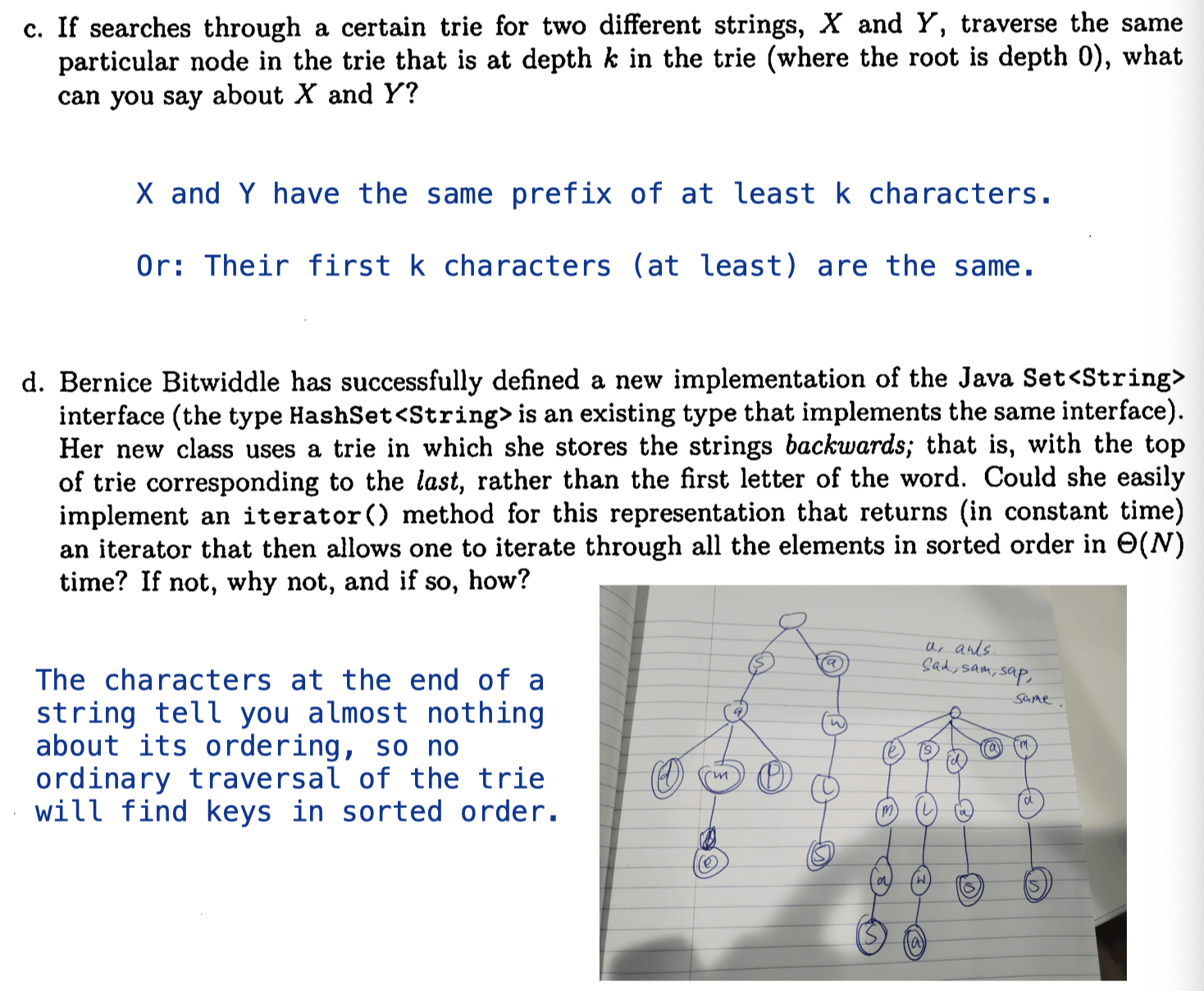## Multi-Dimensional Keys

### Introduction

Suppose we want to perform operations on a set of Body objects in space. For example, perhaps we wanted to ask questions about the Sun bodies (shown as yellow dots below) in our two-dimension image space.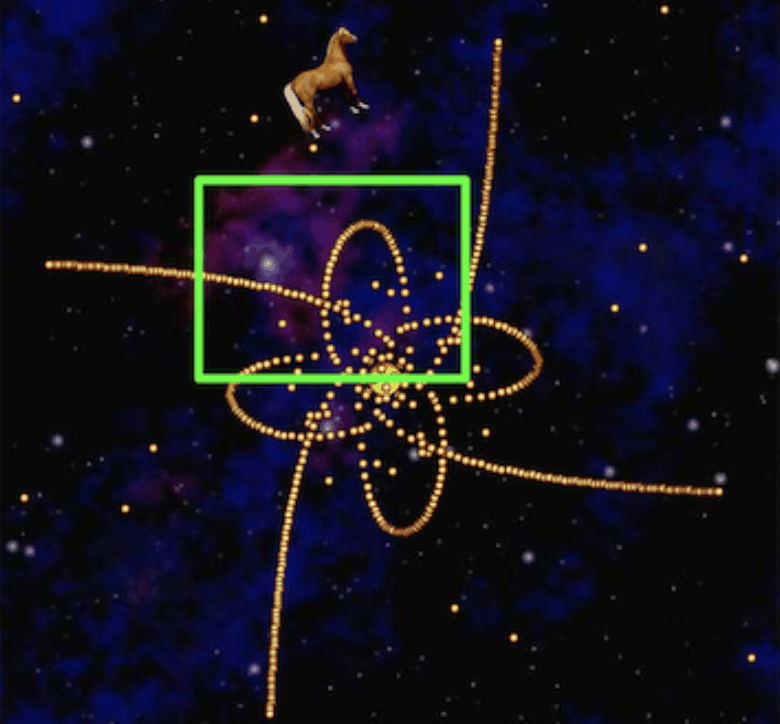Question 1: 2D Range Finding (# suns in a region)

Question 2: Nearest Neighbors (closest to a horse)

HashTable

If we store the suns in a HashTable, the runtime for finding the nearest neighbors is $\Theta(N)$. We need to iterate over all $N$ items in each bucket to check.

Uniform Partitioning

One fix is to ensure that the bucket numbers depend only on position. If we uniformly partition our image space by throwing a 4x4 grid over it, we get nice organized buckets (sometimes called “spatial hashing”).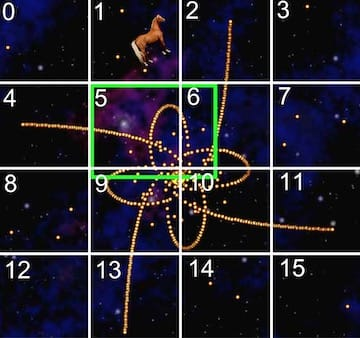So we can put it into a specific bucket by getX() and getY().

For the Question 1, we just need to look into the bucket 1; for the Question 2, we just need to go over the buckets 5, 6, 9, 10.

What is the runtime assuming the suns are evenly spread out?

On average, the runtime will be $16$ times faster than without spatial partitioning, but $\frac{N}{16}$ is still $\Theta(N)$.

But in practice, it does indeed work better!

### X/Y-Based Tree

One key advantage of Search Trees over Hash Tables is that trees explicitly track the order of items. In two (or more) dimensional space, one object might be “less than” another in one dimension, but “greater than” the other in the other dimension.

Say we use the X-based Tree to construct a BST looking only at the x-coordinate (ignore y-coordinate).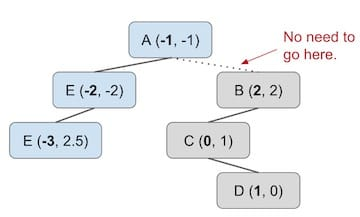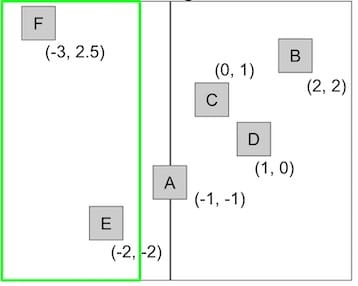We are able to restrict our search space from the entire image space, to just the green rectangle, which is called pruning.

No matter whether we choose the X-based tree representation or the Y-based tree representation, we will always have suboptimal pruning; search in the optimized dimension will be $N\log{N}$, but search in the non-optimized dimension will be $N$ in runtime.

We can solve the problem by splitting in both directions simultaneously.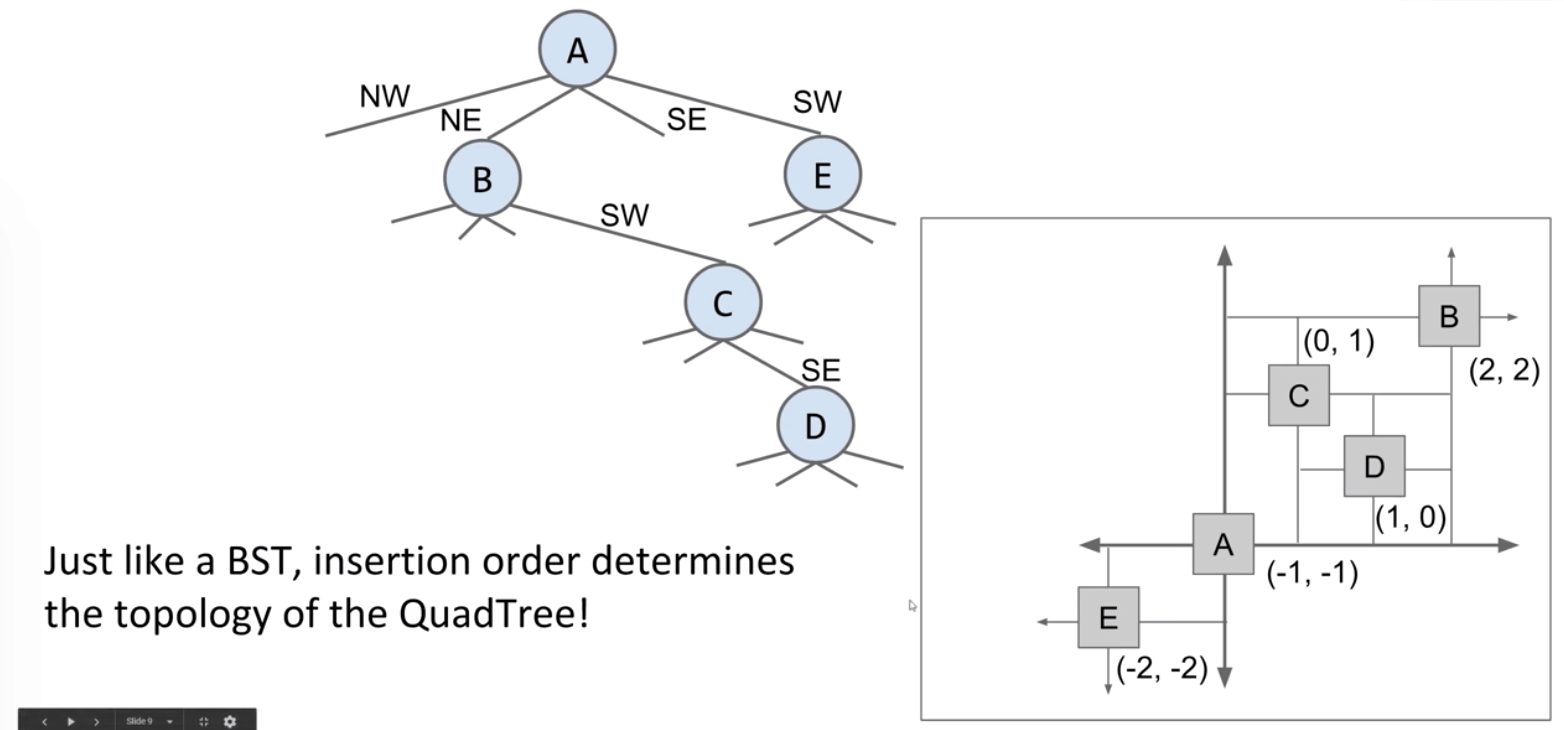QuadTrees are also spatial partitioning in disguise.

Note that just like in a BST, the order in which we insert nodes determines the topology of the QuadTree.

• Earlier approach: Uniform partitioning (perfect grid of rectangles).
• QuadTres: Hierarchical partitioning. Each node “owns” 4 subspaces.
• Space is more finely divided in regions where there are more points.
• Results in better runtime in many circumstances.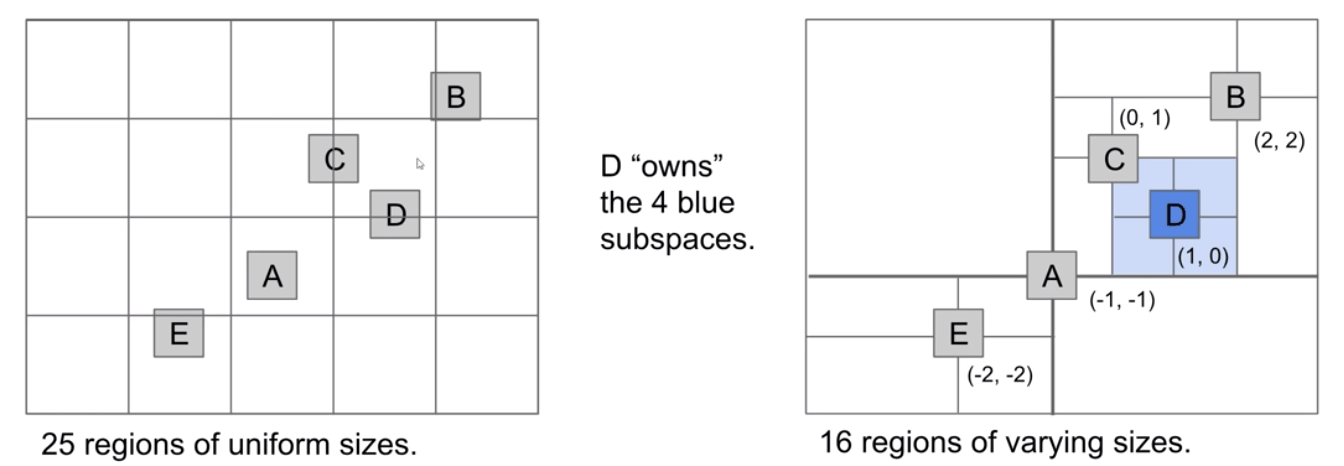How it works in range search?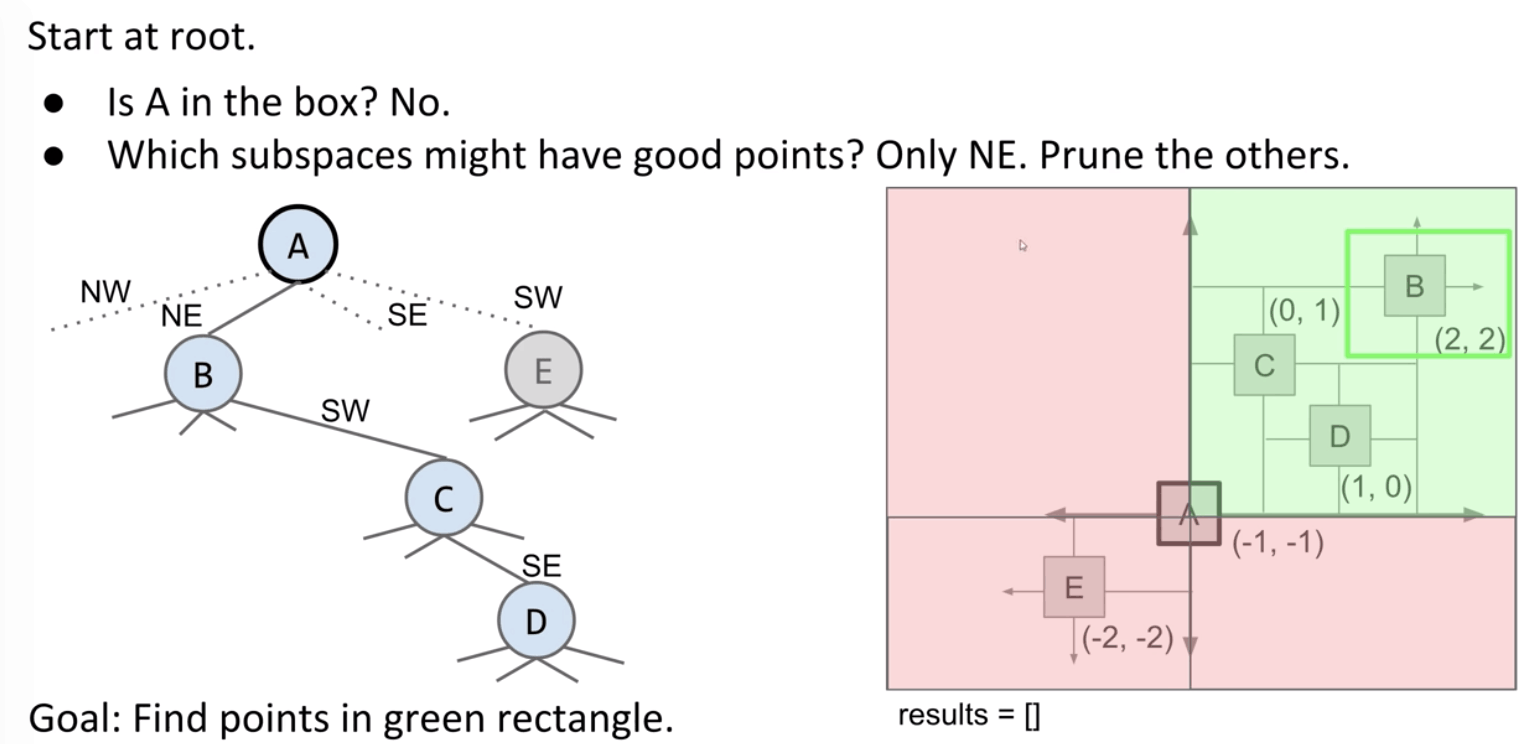Then which subspaces of B might have good points? All four, so explore all four.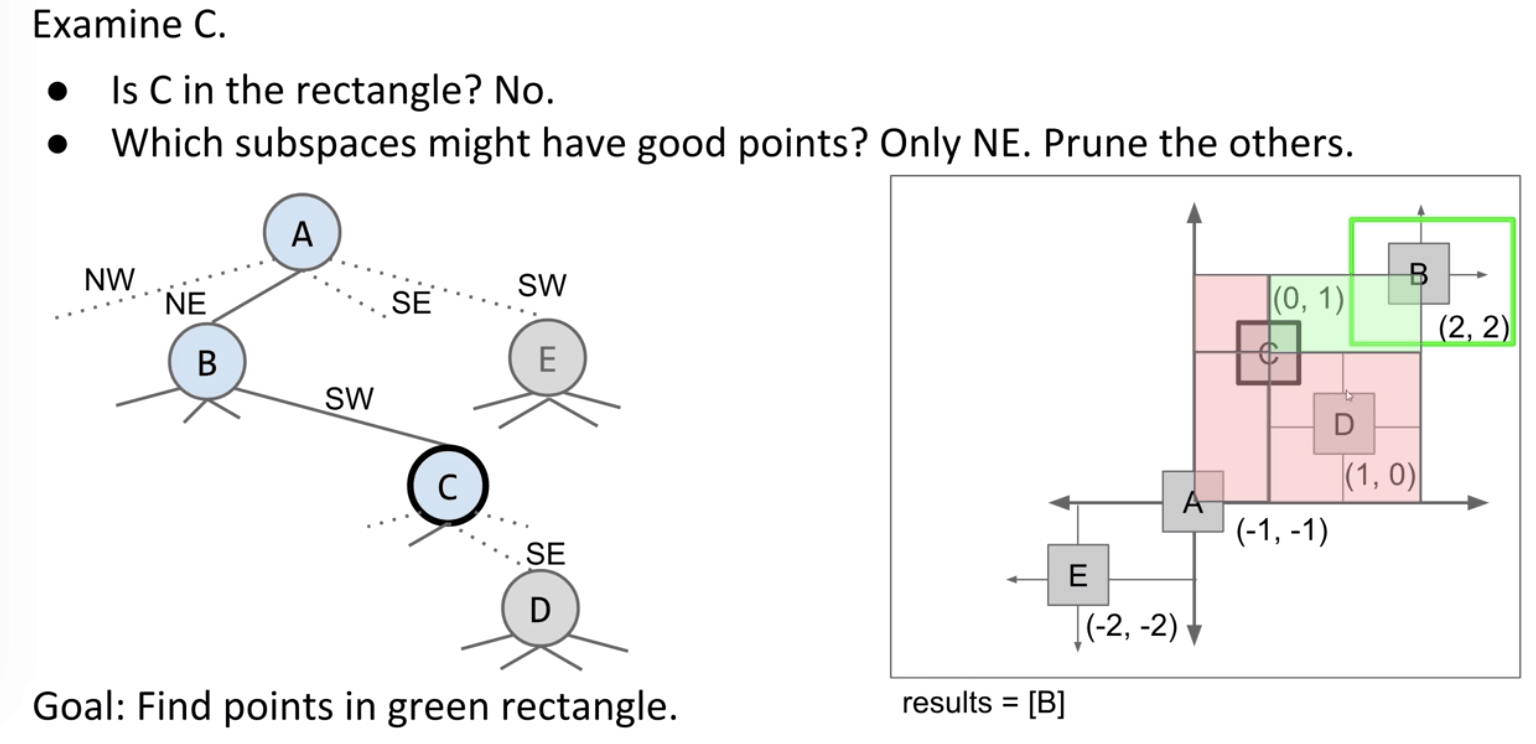As for 3D data, one approach is to use an Oct-Tree or Octree. It is very widely used in practice.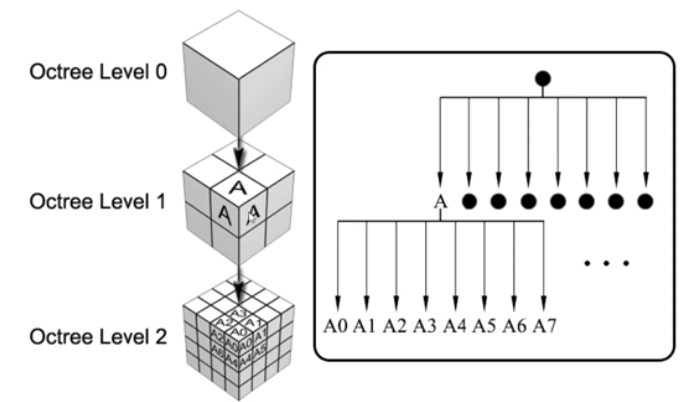### K-D Trees

You may want to organize data on a large number of dimensions like finding songs with features:

• Between 1,000 and 20,000 listens.
• Between 120 to 150 BPM.
• Were recorded after 2004.

In this case, we can use K-D Trees.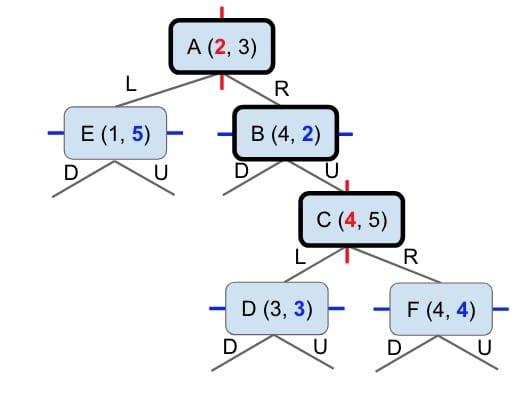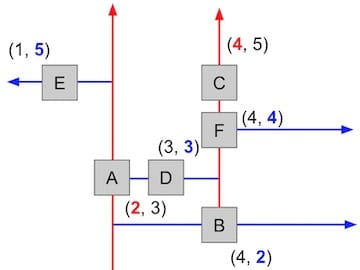Note: When you are running operations on a K-D Tree or quadtree, you should be thinking about the tree structure (1st image) and not the 2-D space (2nd picture) because only the tree holds information about the levels. It is more easily generalized to higher dimensions and keep itself as a binary tree.

For the 3-D case, it rotates between each of the three dimensions every three levels, and so on and so forth for even higher dimensions.

Also, we can break ties by saying that items equal in one dimension should always fall the same way across the border (fall to the right for example).

For a demo on K-D tree insertion, check out these [slides](For a demo on K-D tree insertion, check out these slides).

The takeaway is similar to quadtrees that each point / node controls subspaces. As for K-D trees, each node controls 2 subspaces.

### Nearest Problem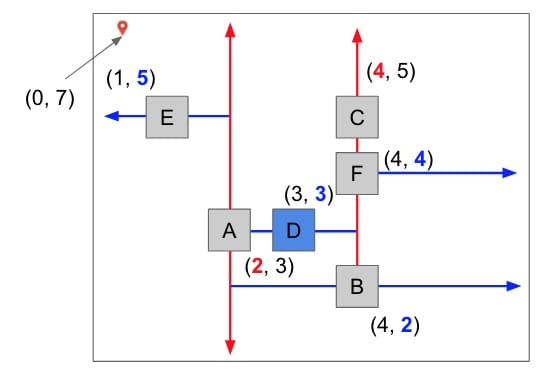• Start at the root and store that point as the “best so far”. Compute its distance to the query point, and save that as the “score to beat”. In the image above, we start at A whose distance to the flagged point is 4.5.
• This node partitions the space around it into two subspaces. For each subspace, ask: “Can a better point be possibly found inside of this space?” This question can be answered by computing the shortest distance between the query point and the edge of our subspace.
• Continue recursively for each subspace identified as containing a possibly better point.
• In the end, our “best so far” is the best point; the point closest to the query point.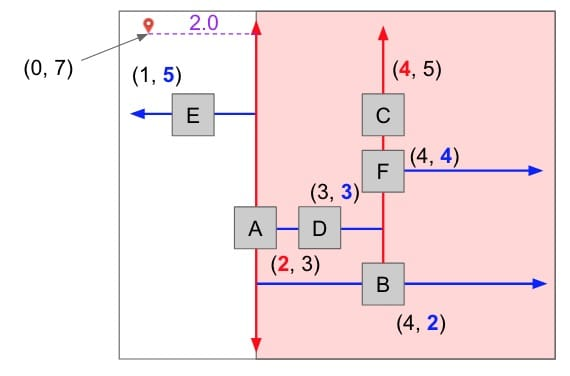For a step by step walkthrough, see these slides. (Very interesting!)

Nearest Pseudocode:

My implementation in Proj2B: link

### Summary

As for the two types of problems, we have the most common approach which is spatial partitioning:

• Uniform Partitioning: Analogous to hashing.
• QuadTree: Generalized 2D BST where each node “owns” 4 subspaces.
• K-D Tree: Generalized K-D BST where each node “owns” 2 subspaces.
• Dimension of ownership cycles with each level of depth in tree. For example, x and y will cycle in 2-D Tree.

Spatial partitioning allows for pruning of the search space.

Application:

• Application #1: Murmuration

Real starlings and simulated starlings. Efficient simulation means “birds” have to find their nearest neighbors efficiently.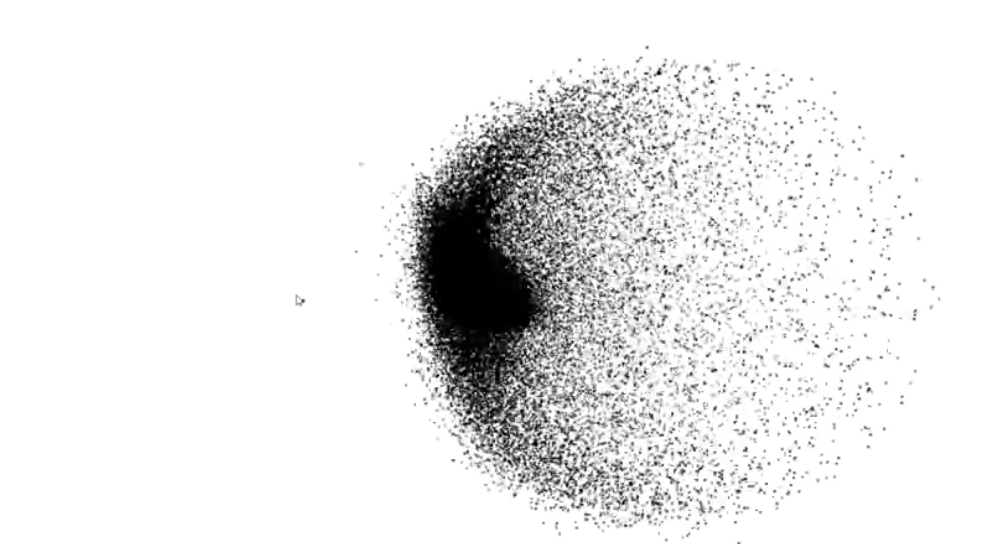• Application #2: NBody Simulation

Every object has to calculate the force from every other object. This is very silly if the force exerted is too small, e.g. individual star in the Andromeda galaxy pulling on the sun.

One optimization is called Barnes-Hut. Basic idea:

• Represent universe as a quadtree (or octree) of objects.
• If a node is sufficiently far away from $X$, then treat that node and all of its children as a single object (e.g. Andromeda).

CommentJunhao Wang
a software engineering cat